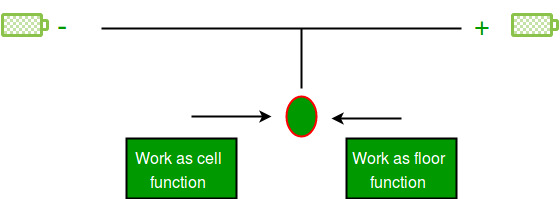Open in App
Not now

# trunc() in Python

• Difficulty Level : Basic
• Last Updated : 22 Jan, 2022

Truncate in Python

There are many built-in modules in python. Out of these module there is one interesting module known as math module which have several functions in it like, ceil, floor, truncate, factorial, fabs, etc.

Out of these functions there is an interesting function called truncate which behaves as a ceiling function for negative number and floor function for positive number.

In case of positive number

## Python3

 `# Python program to show output of floor(), ceil()``# truncate() for a positive number.``import` `math``print` `(math.floor(``3.5``)) ``# floor``print` `(math.trunc(``3.5``)) ``# work as floor``print` `(math.ceil(``3.5``))  ``# ceil`

Output:

```3.0
3
4.0```

In case of negative number

## Python3

 `# Python program to show output of floor(), ceil()``# truncate() for a negative number.``import` `math``print` `(math.floor(``-``3.5``)) ``# floor``print` `(math.trunc(``-``3.5``)) ``# work as ceil``print` `(math.ceil(``-``3.5``))  ``# ceil`

Output:

```-4.0
-3
-3.0```

This is because the ceiling function is used to round up, i.e., towards positive infinity and floor function is used to round down, i.e., towards negative infinity.

But the truncate function is used to round up or down towards zero.
Diagrammatic representation of truncate function:-This article is contributed by Arpit Agarwal. If you like GeeksforGeeks and would like to contribute, you can also write an article and mail your article to review-team@geeksforgeeks.org. See your article appearing on the GeeksforGeeks main page and help other Geeks.